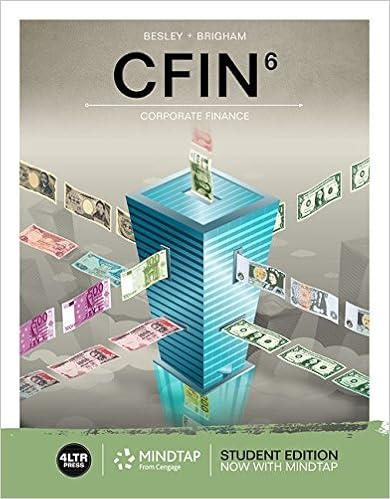Lone star plastics has the following data assets

• 19
• 100% (16) 16 out of 16 people found this document helpful

This preview shows page 14 - 17 out of 19 pages.

We have textbook solutions for you!
The document you are viewing contains questions related to this textbook.The document you are viewing contains questions related to this textbook.
Chapter 12 / Exercise 12-16
CFIN
BesleyExpert Verified
39. Lone Star Plastics has the following data: Assets: P100,000 Interest rate: 8.0% Debt ratio: 40.0% Total asset turnover: Profit margin: 6.0% Tax rate: What is Lone Star’s EBIT? 3.0 40% A. P12,000 C. P30,000 B. P18,000 D. P33,200
40. A firm has a debt/equity ratio of 50%. Currently, it has interest expense of P500,000 on P5,000,000 of total debt outstanding. Its tax rate is 40%. If the firm’s ROA is 6%, by how many percentage points is the firm’s ROE greater than its ROA?
41. Watson Corporation computed the following items from its financial records for the year just ended: Price-earnings ratio 12 Payout ratio .6 Asset turnover .9 The dividend yield on Watson’s common stock is
42. Lombardi Trucking Company has the following data: Assets: P10,000 Interest rate: 10.0%
We have textbook solutions for you!
The document you are viewing contains questions related to this textbook.The document you are viewing contains questions related to this textbook.
Chapter 12 / Exercise 12-16
CFIN
BesleyExpert Verified
Debt ratio: 60.0% Total asset turnover: Profit margin: 3.0% Tax rate: What is Lombardi’s TIE ratio? 2.0 40%
43. Miller and Rogers Partnership has P3 million in total assets, P1.65 million in equity, and a P500,000 capital budget. To maintain the same debt-equity ratio, how much debt should be incurred? A.P 50,000 C. P275,000 B. P225,000 D. P450,000
44. Standard Company’s bonds have a provision which stipulates that the ratio of senior debt to total assets will never rise above 45%. The company is at the limit of that ratio and it wishes to issue still another P25 million in senior debt. How much additional equity capital must it raise to comply with this restrictive provision?
45. India Oats pays dividends of P0.62 per quarter, and has annual earnings per share of P2.80. What is India Oats’s dividend yield and dividend payout ratio for 2000, respectively, if its recent market price is P30.00 and its average market price was P28.00?
46. Assume Meyer Corporation is 100% equity financed. Calculate the return on equity, given the following information: (1) Earnings before taxes = P1,500 (2) Sales = P5,000 (3) Dividends payout ratio = 60% (4) Total asset turnover = 2.0 (5) Tax rate = 30%
47. Beatnik Company has a current ratio of 2.5 and a quick ratio of 2.0. If the firm experienced P2 million in sales and sustains an inventory turnover of 8.0, what are the firm’s current assets? A. P1,000,000 C. P1,500,000 B. P500,000 D. P1,250,000
48. Hanson Corporation’s present year ROE remained at last year’s 14% level, while the profit margin was reduced from 8% to 4% and the leverage ratio increased from 1.2 to 1.5. The effects on asset turnover were to
•••